# Unsteady Hydromagnetic Forced Convective Heat Transfer Flow of a Micropolar Fluid Along a Porous Wedge with Convective Surface Boundary Condition

Unsteady Hydromagnetic Forced Convective Heat Transfer Flow of a Micropolar Fluid Along a Porous Wedge with Convective Surface Boundary Condition

M. S. Alam Tarikul Islam M. M. Rahman*

Dept of Math, Jagannath University, Dhaka-1100, Bangladesh;

Dept of Math and Stat, College of Sci, Sultan Qaboos University, PO Box 36, PC 123 Al-Khod, Muscat, Sultanate of Oman;

Corresponding Author Email:
mansurdu@yahoo.com
Page:
115-122
|
DOI:
https://doi.org/10.18280/ijht.330219
N/A
|
Accepted:
N/A
|
Published:
30 June 2015
| Citation

OPEN ACCESS

Abstract:

In this paper we analyze unsteady two dimensional hydromagnetic forced convective heat transfer flow of a viscous incompressible micropolar fluid along a permeable wedge with convective surface boundary condition. The potential flow velocity has been taken as a function of the distance x and time t. The governing time dependent non-linear partial differential equations have been reduced to a set of non-linear ordinary differential equations by introducing a new class of similarity transformations. Comparisons with previously published work are performed, and the results are found to be in excellent agreement. The resulting local similarity equations for unsteady flow have been solved numerically by applying Nachtsheim-Swigert shooting iteration technique along with sixth order Runge-Kutta integration scheme.Numerical results in the form of non-dimensional velocity, microrotation and temperature profiles are presented graphically and discussed for different material parameters entering into the analysis. The effects of the pertinent parameters on the local skin- friction coefficient, plate couple-stress and the rate of heat transfer are also displayed in tabulated form and discussed them from the physical point of view. The obtained numerical results show that the rate of heat transfer increases with the increase of the unsteadiness parameter and decreases with the increase of the surface convection parameter.

Keywords:

Heat transfer, Unsteady wedge flow, Micropolar fluid, Convective surface.

1. Introduction

A micropolar fluid is the fluid with internal structures in which coupling between the spin of each particle and the macroscopic velocity field is taken into account. The classical theories of continuum mechanics are inadequate to explain the microscopic manifestations of such complex hydrodynamic behavior. The dynamics of micropolar fluids, originated from the theory of Eringen [1, 2] has been a popular area of research due to their applications in a number of processes that occurs in industry. Such applications include the flow of exotic lubricants, colloidal suspensions, solidification of liquid crystals, extrusion of polymer fluids, cooling of metallic plate in bath, animal bloods, body fluids and many other situations. An excellent review of micropolar fluids and their applications was given by Ariman et al. . Wang  studied the coupling condition with mixed convection of micropolar fluid past a vertical plate.  Kim  and Kim and Kim have considered the steady boundary layer flow of a micropolar fluid along a wedge with constant surface temperature and constant surface heat flux, respectively. Ishak et al.  studied the steady laminar MHD boundary layer flow along a wedge immersed in an incompressible micropolar fluid in the presence of a variable magnetic field.  Rahman and his coworkers have studied and reported results on micropolar

fluids for various flow and thermal conditions in different geometries [8-16]. Elbashbeshy et al.  studied the effect of magnetic field on boundary layer flow over an unsteady stretching surface in a micropolar fluid. Eldabe et al.  studied effect of thermal radiation on heat transfer over an unsteady stretching surface in a micropolar fluid with variable heat flux. Recently Alam and Chapal  introduced a new similarity approach for an unsteady two dimensional forced convective flow of a micropolar fluid along a wedge. Unlike the works of Alam and Chapal , the objective of the present paper is to obtain a local similarity solution of an unsteady two dimensional hydromagnetic forced convective heat transfer flow of a viscous incompressible micropolar fluid along a porous wedge with convective surface boundary condition.

2. Formulation of the Problem

2.1 Flow analysis

We consider an unsteady laminar boundary layer flow past a porous wedge in an electrically conducting micropolar fluid in the presence of a magnetic field B. The magnetic Reynolds number of the flow is taken to be small enough so that the induced magnetic field is assumed to be negligible in comparison with applied magnetic field so that B = (0, B0, 0), where B0 is the uniform magnetic field acting normal to the wedge surface. The angle of the wedge is given by $\Omega=\beta \pi$. The flow is assumed to be in the x-direction which is taken along a direction of the wedge and the y-axis normal to it. Fluid suction/injection is imposed at the wedge surface and considering the suction hole size constant.It is also assumed that the lower surface of the wedge is heated by convection from a hot fluid of temperature $T_{f}$  which provides a heat transfer coefficient $h_{f}$ . The flow configurations and coordinate system are shown in Figure 1.

Under the above assumptions and usual boundary layer approximation, the governing equations for this problem can be written as (see also Alam and Chapal ):

## 1.png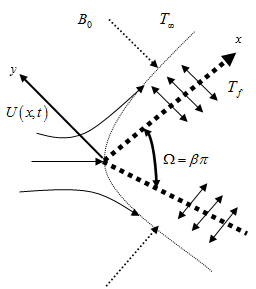Figure 1. Flow configurations and coordinate system

$\frac{\partial u}{\partial x}+\frac{\partial v}{\partial y}=0$   (1)

$\frac{\partial u}{\partial t}+u \frac{\partial u}{\partial x}+v \frac{\partial u}{\partial y}=\frac{\partial U}{\partial t}+U \frac{\partial U}{\partial x}+v_{a} \frac{\partial^{2} u}{\partial y^{2}} +\frac{S}{\rho} \frac{\partial N}{\partial y}-\frac{\sigma B_{0}^{2}}{\rho_{\infty}}(u-U)$    (2)

$\frac{\partial N}{\partial t}+u \frac{\partial N}{\partial x}+v \frac{\partial N}{\partial y}=\frac{v_{s}}{\rho j} \frac{\partial^{2} N}{\partial y^{2}} -\frac{S}{\rho j}\left(2 N+\frac{\partial u}{\partial y}\right)$   (3)

$\frac{\partial T}{\partial t}+u \frac{\partial T}{\partial x}+v \frac{\partial T}{\partial y}=\frac{\kappa}{\rho c_{p}} \frac{\partial^{2} T}{\partial y^{2}}$   (4)

where $u, v$ are the velocity components along $x, y$ co-ordinates respectively, $t$ is the time, $v_{a}=\frac{\mu+S}{\rho}$ is the apparent kinematic viscosity, $v_{s}=\left(\mu+\frac{S}{2}\right) j$ is the microrotation viscosity (or spin-gradient viscosity),  $\mu$ is the coefficient of dynamic viscosity, $S$ is the microrotation coupling coefficient (also known as the coefficient of gyro-viscosity or as the vortex viscosity), $\rho$ is the density of the fluid, $N$ is the microrotation component normal to the $x y$-plane, $j$ is the micro-inertia density, $T$ is the temperature of the fluid within the boundary layer,  $T_{\infty}$ is the free stream temperature, $c_{p}$ is the specific heat of the fluid at constant pressure and  $\kappa$ is the thermal conductivity.

2.2 Boundary conditions

The boundary conditions for the above problem are

(i) On the surface of the wedge $(y=0)$:

$u=0, v=\pm v_{w}(x), N=-n \frac{\partial u}{\partial y},-\kappa \frac{\partial T}{\partial y}=h_{f}\left(T_{f}-T\right)$  (5a)

(ii) Matching with the free stream ($y \rightarrow \infty$):

$u=U(x, t)=\frac{v x^{m}}{\delta^{m+1}}, N=0, T=T_{\infty}$    (5b)

where $V_{w}$ (x, t) represents the suction/ injection velocity at the  porous surface where its sign indicates suction (<0) or injection (>0) and $U(x, t)$ is the potential velocity generated by the pressure gradient.

The subscripts $f$ refer to the surface condition and the exponent $m$ is known as Hartee pressure gradient parameter which is a function of the wedge angle parameter $\beta$.

The value of microrotation parameter $n=0$ results $N=0$ which represents no-spin condition i.e. the microelement in concentrated particle flow-close to the wall are not able to rotate as stated by Jena and Mathur . The case $n=\frac{1}{2}$  physically represents the vanishing of the anti-symmetric part of the stress tensor and represents weak concentrations of the micro-elements of the micropolar fluid at the solid surface. For this case Ahmadi  suggested that in a fine particle suspension the particle spin is equal to the fluid velocity at the wall. The case corresponding to $n=1$  represents the turbulent boundary layer flows (see Peddison and McNit ).

The potential flow velocity $U(x, t)$ for the wedge flow has been taken as follows (see also Sattar, Rahman et al. [24-26])

$U(x, t)=\frac{v x^{m}}{\delta^{m+1}}$     (6)

where $\delta$ is a time dependent length scale as

$\delta=\delta(t)$    (7)

The exponent m is a function of the wedge angle parameter $\beta$ such as $m=\frac{\beta}{2-\beta} \geq 0$ .

The wedge angle parameter $\beta$  is a measure of pressure gradient, and so a positive value of  $\beta$  indicates a negative (or favorable) pressure gradient. It is to be mentioned that $\beta$  may be negative in which case the flow is considered to be decelerated. In the present work we considered only the accelerated flows i.e. $\beta>0$.

2.3 Nondimensionalization

In order to obtain similarity solution of the above system of equations (1)-(4) under the boundary conditions (5) we introduce the following non-dimensional variables (see also Alam and Chapal ):

$\eta=y \sqrt{\frac{m+1}{2}} \sqrt{\frac{x^{m-1}}{\delta^{m+1}}}, \psi=v \sqrt{\frac{2}{m+1}} \sqrt{\frac{x^{m+1}}{\delta^{m+1}}} f(\eta)$

$\theta(\eta)=\frac{T-T_{\infty}}{T_{f}-T_{\infty}}, N=v \sqrt{\frac{m+1}{2}} \frac{x^{\frac{3 m-1}{2}}}{\delta^{\frac{3 m+3}{2}}} g(\eta)$     (8)

where η is the similarity variable and ψ is the stream function  that satisfies the continuity equation (1) such that $u=\frac{\partial \psi}{\partial y}$ and $v=-\frac{\partial \psi}{\partial x}$.

Now using equations (6)-(8) into equations (2)-(4) we obtain the following nonlinear ordinary differential equations:

$(1+\Delta) f^{\prime \prime \prime}+f f^{\prime \prime}+\frac{2 m}{m+1}\left(1-f^{\prime 2}\right)+\Delta g^{\prime}$

$-K\left[2-2 f^{\prime}-\eta f^{\prime \prime}\right]-\frac{2 M}{m+1}\left(f^{\prime}-1\right)=0$   (9)

$b g^{\prime \prime}-\frac{2 \Delta B}{m+1}\left(f^{\prime \prime}+2 g\right)+f g^{\prime}-\frac{3 m-1}{m+1} f^{\prime} g+K\left(3 g+\eta g^{\prime}\right)=0$     (10)

$\theta^{\prime \prime}+\operatorname{Pr} f \theta^{\prime}+K \operatorname{Pr} \eta \theta^{\prime}=0$  (11)

where primes denote differentiations with respect to the variable $\eta$ only.

The dimensionless parameters which appear in the above equations are as follows: $\Delta=\frac{S}{\rho v}$ is the vortex viscosity parameter, $K=\frac{\delta^{m}}{v x^{m-1}} \frac{d \delta}{d t}$ is the unsteadiness parameter, $M=\frac{\sigma B_{0}^{2}}{\rho U} \frac{\delta^{m+1}}{x^{m-1}}$ is the magnetic field parameter,$b=\frac{v_{s}}{\rho v j}$ is the spin gradient viscosity parameter, $B=\frac{\delta^{m+1}}{j x^{m-1}}$ is the local micro-inertia density parameter and $\mathrm{Pr}=\frac{\mu c_{p}}{\kappa}$ is the Prandtl number.

Further, we suppose that  $K=\frac{c}{x^{m-1}}$ , where c is a constant so that

$c=\frac{\delta^{m}}{v} \frac{d \delta}{d t}$   (12)

Integrating (12) we obtain

$\delta=[c(m+1) v t]^{1 / m+1}$    (13)

Now taking $c=2$ and $m=1$ in equation (13) we obtain

$\delta=2 \sqrt{v t}$    (14)

The value $m=1$ represents the condition for a flat plate through which the flow is termed as stagnation point flow. It appears from equation (14) that the length scale $\delta=2 \sqrt{v t}$ for the ordinate is similar to Stokes’  for an unsteady parallel flow but the form of $\delta(t)$ was initially developed by Sattar and Hossain  for an unsteady one-dimensional boundary layer problem. The characteristic length scale $\delta(t)$ defined particularly in (14) physically related to the boundary layer thickness that can be found in the book by Schlichting .

The transformed boundary conditions are given by

$f=f_{w}, f^{\prime}=0, g=-n f^{\prime \prime}, \theta^{\prime}=B i \sqrt{\frac{2}{m+1}}(\theta-1) a t \quad \eta=0$

$f^{\prime}=1, \quad \theta=0, \quad g=0$  as $\quad \eta \rightarrow \infty$    (15)

where $f_{w}=\pm v_{w}(x, t)\left(\frac{2}{m+1}\right)^{\frac{1}{2}} \frac{\delta^{\frac{m+1}{2}}}{v x^{\frac{m-1}{2}}}$ is the wall mass transfer coefficient which is positive ( $f_{w}$ > 0) for suction and negative ( $f_{w}$ < 0 ) for injection, $B i=\frac{h_{f} x}{\kappa}(\mathrm{Re})^{-\frac{1}{2}}$ is Biot number or, the surface convection parameter and $\mathrm{Re}=\frac{U x}{v}$ is the local Reynolds number.

2.4 Important physical parameters

The parameters of engineering interest for the present problem are the local skin friction coefficient (rate of shear stress), local plate couple stress and local Nusselt number (rate of heat transfer) which are given in the following expressions:

$\frac{1}{2} C_{f x} \operatorname{Re}^{\frac{1}{2}}=\sqrt{\frac{m+1}{2}}[1+(1-n) \Delta] f^{\prime \prime}(0)$    (16)

$M_{w x}=\frac{b(m+1) g^{\prime}(0)}{(1+\Delta) B}$    (17)

$N u_{x} \operatorname{Re}^{-\frac{1}{2}}=B i(1-\theta(0))$    (18)

3. Numerical Solutions

The set of ordinary differential equations (9)-(11) is highly nonlinear and coupled and therefore the system cannot be solved analytically. The  nonlinear ordinary differential equations (9)-(11) along with the corresponding boundary conditions (15) have been solved numerically by applying sixth order Runge-Kutta integration scheme together with Nachtsheim-Swigert  shooting iteration technique (for detailed discussion of the method see also Alam et al.). The solutions are affected by magnetic field parameter $M$, suction/injection parameter $f_{w}$, wedge angle parameter (or, Hartee pressure gradient parameter) $m$, unsteadiness parameter $K$ , microrotation parameter $n$ , vortex viscosity parameter $\Delta$ , spin-gradient viscosity parameter $b$, micro-inertia density parameter $B$ , Prandtl number $\operatorname{Pr}$ and Biot number $B i$ . A step size of $\Delta \eta=0.001$ was selected to be satisfactory for a convergence criterion of 10-6 in all cases. The value of $\eta_{\infty}$ was found to each iteration loop by the statement$\eta_{\infty}=\eta_{\infty}+\Delta \eta$. The maximum value of $\eta_{\infty}$ to each group of parameters $\Delta, m, K, n, b, B, \operatorname{Pr}, M, f_{w}$，$B i$ determined when the value of the unknown boundary conditions at $\eta=0$ does not change to successful loop with an error less than 10-6.

3.1 Testing of code

To assess the accuracy of the present code, we calculated the values of $f^{\prime}(\eta)$ and $f^{\prime \prime}(\eta)$ for the Falkner-Skan boundary layer equation when $\Delta=m=K=M=0$ for different values of $\eta$. FromTable 1 we observe that the data produced by the present code and that of White  are in excellent agreement. This gives us confidence to use the present numerical method.

Table 1. Comparison of the present numerical results with White for Falkner-Skan boundary layer flow when $\Delta=m=K=M=0$.

 h $f^{\prime}(\eta)$ $f^{\prime}(\eta)$ $f^{\prime \prime}(\eta)$ $f^{\prime \prime}(\eta)$ Present work White  Present work White  0.0 0.0000000 0.000000 0.46962969 0.46960 1.0 0.4606599 0.46063 0.43440208 0.43438 2.0 0.8173262 0.81669 0.25567169 0.25567 3.0 0.9690878 0.96905 0.06770646 0.06771 4.0 0.9978003 0.99777 0.00687331 0.00687 5.0 0.9999657 0.99994 0.00025775 0.0002
4. Results and Discussion

Numerical calculations have been carried out for different values of the physical parameters such as magnetic field parameter $M$, pressure gradient parameter $m$, unsteadiness parameter $K$ , spin-gradient viscosity parameter $b$, micro-inertia density parameter $B$ and Biot number $B i$ keeping Prandtl number $\operatorname{Pr}$ as fixed. Here we have considered human blood as the micropolar fluid. At $T=310 K$ (human body temperature); the value of $\rho=1.05 \times 10^{3} \mathrm{kg} / \mathrm{m}^{3}$,$\mu=3.2 \times 10^{-3} \mathrm{kg} / \mathrm{ms}$ ,$c_{p}=14.65 J / \mathrm{kg} K$ and $\kappa=2.2 \times 10^{-3} \mathrm{J} / \mathrm{ms} \mathrm{K}$ . Thus, the value of the Prandtl number becomes $\mathrm{Pr}=\frac{\mu c_{p}}{\kappa}$= 21 (see also Chato and Valvano et al. ). Since the experimental data of the other physical parameters are almost not available therefore in the numerical simulations the choice of the values of the parameters was dictated by the values chosen by the previous investigators. In the simulations the default values of the parameters are considered to be$\Delta=2.0, K=0.5, m=0.2, B=0.5$，$b=2.0, \quad n=0.5, \quad M=1.0, f_{w}=0.5$and $B i=0.5$ unless otherwise specified.

The effects of the magnetic field parameter $M$ on the dimensionless velocity, microrotation and temperature profiles across the boundary layer are displayed in Figures 2(a)-(c) respectively.  It can be seen from Figure 2(a) that the fluid velocity profiles increase monotonically with the increase of magnetic field parameter $M$. On the other hand, the results also show that the velocity boundary layer thickness decreases with the increase of  the magnetic field parameter $M$ which in turn increases the velocity gradient at the surface ($\eta=0$), and hence produce an increase in skin friction coefficient. This is expected, since the application of a magnetic field moving with the free stream has the tendency to induce a motive force, which increases the motion of the fluid and hence increase the surface friction force. From Figure 2(b) we see that the dimensionless microrotation profiles remain negative. From this figure we observe that the microrotation profiles are found to decrease with the increase of the magnetic field parameter $M$ within some domain $\eta \leq \eta_{\text {critical }}$ and increase within the domain \eta>\eta_{\text {critical}}. It is also found that at the surface of the wedge the maximum microrotation (angular velocity) of the microconstituents is found to be  -0.409331, -0.597651, -0.732561, and -0.842062 for M= 0.0, 1, 2.0, 3.0 respectively. Thus, the microrotation of the microconstituentsat the surface of the wedge decreases 46.01% when M increase from 0.0 to 1.0 whereas the corresponding decrease is 14.95%, when M increase from 2.0 to 3.0.  From Figure 2(c) we also see that the temperature profile slightly decrease with the increase of magnetic field parameter M. Therefore, an applied magnetic field can be used to control the flow, rotation of micro-constituents and heat transfer characteristics.

Figures 3(a)-(c), respectively, show the velocity, microrotation and temperature profiles for different values of the pressure gradient parameter m. The value m=0 corresponds to wedge angle of zero degree i.e. flat plate and m=1 corresponds to the wedge angle of 180 degrees i.e. stagnation point flow. From Figure 3(a) it is clear that the fluid velocity within the boundary layer increases monotonically with the increasing values of the pressure gradient parameter m.The results also show that the velocity profiles become steeper for large values of the pressure gradient parameter m.   From Figure 3(b) we observe that the microrotation profiles decrease with the increase of the values of the pressure gradient parameter m when $\eta \leq \eta_{\text {critical }}$  . For $\eta>\eta_{\text {critical }}$ a reversed effect is observed in the upper portion of the boundary layer. It is also found that at the surface of the wedge the maximum microrotation (angular velocity) of the microconstituents is found tobe -0.450692, -0.512274, -0.568747, and -0.629646 for m= 0.0, 0.33, 1.0, 4.0  respectively. Thus the microrotation profiles decrease by13.66% when m increase from 0.0 to 0.33 whereas the corresponding decrease is 10.71%, when m increases from 1.0 to 4.0. Figure 3(c) shows that the non-dimensional temperature profiles within the boundary layer for different values of the pressure gradient parameter m. From this figure we see that the temperature profiles decrease with the increasing values of the pressure gradient parameter m. It is also found that at the surface of the wedge the maximum temperature of the fluid is found to be 0.062285, 0.052663, 0.043319, and 0.027814 for $m=0.0,0.33,1.0,4.0$ respectively. Thus, the temperature of the fluid at the surface of the wedge decreases by15.45% when m increases from 0.0 to 0.33 whereas the corresponding decrease is 35.79 %, when m increases from 1.0 to 4.0.

## 2a.png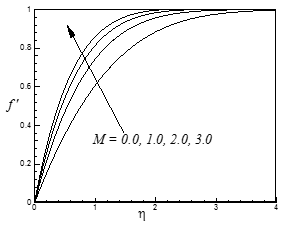(a)

## 2b.png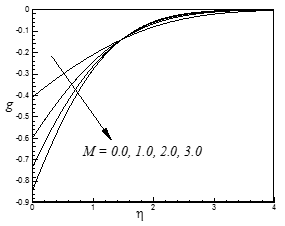(b)

## 2c.png(c)

Figure 2. Variation of dimensionless (a) velocity, (b) microrotation and (c) temperature profiles for different values of M.

## 3a.png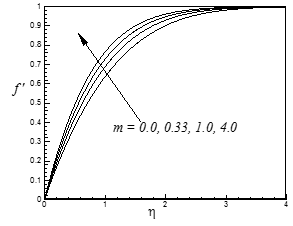(a)

## 3b.png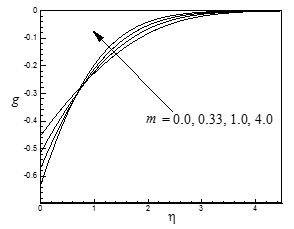(b)

## 3c.png(c)

Figure 3. Variation of dimensionless (a) velocity, (b) microrotation and (c) temperature profiles for different values of m.

The effects of the unsteadiness parameter $K$ on the velocity, microrotation and temperature profiles are shown in Figure 4(a)-(c) respectively. From Figure 4(a) we observe that the velocity profiles decrease with the increasing values of the unsteadiness parameter $K$ for $\eta \leq \eta_{\text {critical }}$ . But for $\eta>\eta_{\text {critical }}$, a reverse trend is observed. This figure also shows that a backflow phenomenon occurs for $0<K<K_{\text {critical}}$ (not precisely determined).  From Figure 4(b), we observe that microrotation of the blood corpuscles increases with theincrease of the unsteadiness parameter K in the vicinity of the surface of the wedge. But far away from the surface of the wedge where kinematic viscosity dominates the flow microrotation profiles overlap and decrease with the increase of unsteadiness parameter $K$. This is consistent with the work of Rahman and Sattar . From this figure, we also found that at the surface of the wedge the maximum microrotation of the microconstituents is -0.588525, -0.416873, -0.214564 and -0.004872 for $K=0.5,1.0,2.0,3.0$ respectively. Thus, the microrotation of the blood corpuscles at the surface of the wedge increases 29.17% when $K$ increases from 0.5 to 1.0 whereas the corresponding increase is 97.72%, when $K$ increases from 2.0 to 3.0. Figure 4(c) shows that the non-dimensional temperature profiles within the boundary layer for different values of the unsteadiness parameter $K$. From this figure we see that the temperature profiles decrease with the increasing values of the unsteadiness parameter $K$. It is also found that at the surface of the wedge the maximum temperature of the fluid is 0.055184, 0.048692, 0.044612, and 0.041635 for $K=0.5,1.0,2.0,3.0$ respectively. The temperature of the micropolar fluid at the surface of the wedge decreased by 11.76% when $K$ was increased from 0.5 to 1.0 whereas the corresponding decrease was 6.67%, when $K$ increased from 2.0 to 3.0.  Therefore the unsteadiness parameter $K$ controls the flow, rotation of the micro-constituents and heat transfer characteristics.

## 4a.png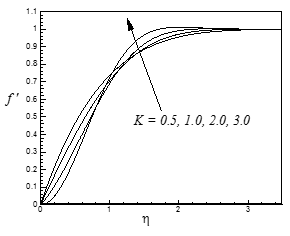(a)

## 4b.png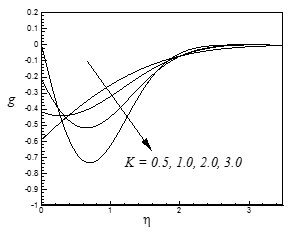(b)

## 4c.png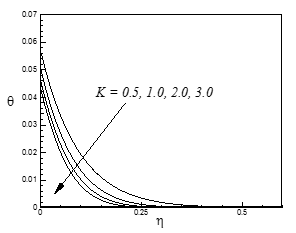(c)

Figure 4: Variation of dimensionless (a) velocity,(b) microrotation and (c) temperature profiles for different values of K.

## 5a.png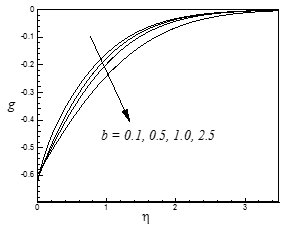(a)

## 5b.png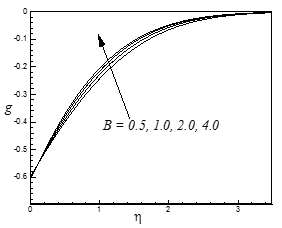(b)

## 5c.png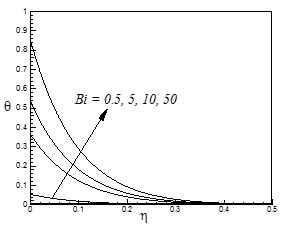(c)

Figure 5: Variation of dimensionless microrotation profiles for different values of (a) b, (b) B and (c) temperature profiles for different values of Bi.

The effects of the spin gradient viscosity parameter b and the micro-inertia density parameter $B$ on the dimensionless microrotation profiles have been displayed in Figure 5(a)-(b) respectively.

From Figures 5(a)-(b) we observe that the microrotation profiles are found to decrease with the increasing values of $b$ whereas it increases with the increasing value of $B$.

The effect of the Biot number (surface convection parameter) $B i$ on the temperature profiles against $\eta$ is displayed in Figure 5(c). From this figure we observe that the temperature profiles within the boundary layer increase with the increase of the Biot number $B i$. The surface convection parameter or Biot number is a ratio of the hot fluid side convection resistance to the cold fluid side convection resistance on a surface. For fixed cold fluid properties and fixed free stream velocity, the surface convection parameter $B i$ at any $x$ station is directly proportional to the heat transfer coefficient, $h_{f}$ associated with the hot fluid. The thermal resistance on the hot fluid side is inversely proportional to $h_{f}$ . As Bi increases, the hot fluid side convection resistance decreases and consequently, the surface temperature increases. This is consistent with the work of Rahman and Sattar . It is also found that at the surface of the wedge the maximum temperature of the fluid is 0.050807, 0.365087, 0.534893 and 0.851372 which occur for $B i$= 0.5, 5.0, 10.0 and 50.0 respectively. Therefore at the surface of the wedge the fluid temperature increases by 618.57% when $B i$ increases from 0.5 to 5.0 whereas the corresponding increase is 59.17% when $B i$ increases from 10.0 to 50.0. We further notice that throughout the boundary layer the temperature profiles decrease monotonically with the increase of $\eta$  . From this figure it is also confirmed that for large values of $B i$ i.e. $B i \rightarrow \infty$ , the temperature profile attains its maximum value 1: thus the convective boundary condition become the prescribed surface temperature case.

Table 2. Numerical values of the local skin-friction coefficient, plate couple stress and the Nusselt number for different values of $M$ and $B i$.

 $M$ Bi $f^{\prime \prime}(0)$ $g^{\prime}(0)$ $-\theta^{\prime}(0)$ 1.0 0.5 5.0 10 50 1.1794999 1.1794999 1.1794999 1.1794999 0.40830937 0.40830937 0.40830937 0.40830937 0.943018148 0.623344324 0.452796102 0.141995073 0.0 0.5 1.0 2.0 0.5 0.73219433 0.98716544 1.17949990 1.47826448 0.17487508 0.30400641 0.40830937 0.57970315 0.942712748 0.942891598 0.943018148 0.944021746

Finally, the combined effects of magnetic field parameter $M$ and Biot number (surface convection parameter) $B i$ on the local skin-friction coefficient ($f^{\prime \prime}(0)$), local plate couple stress ($g^{\prime}(0)$) and the local Nusselt number($-\theta^{\prime}(0)$) have been shown in Table-2. From this table we see that the local skin-friction coefficient increases with an increasing value of the magnetic field parameter $M$ when the Biot number (surface convection parameter) $B i$ is fixed. On the other hand from this table we see that for a fixed value of the magnetic field parameter $M$,  the rate of heat transfer decreases with an increasing values of the Biot number (surface convection parameter) Ві.

5. Conclusions

In this paper we have studied the problem of unsteady hydromagnetic forced convective heat transfer flow of a micropolar fluid along a permeable wedge with convective surface boundary condition. With the help of these similarity transformations, the governing boundary-layer equations are reduced to ordinary differential equations, which are then solved numerically by applying Nachtsheim-Swigert shooting iteration method. In the numerical computations, we have considered human blood as the micropolar fluid with $\mathrm{Pr}=21$. Comparison with previously published work was performed and the results were found to be in excellent agreement. The numerical results have been presented in the form of graphs From the numerical computations the following major findings can be concluded:

• Magnetic field and unsteadiness significantly control the flow, rotation of micro-constituents and heat transfer characteristics of a micropolar fluid.

• The local skin-friction coefficient increases with the increasing values of the magnetic field parameter.

• The plate couple stress increases with the increase values of the magnetic field parameter.

• The local Nusselt number increases with the increasing value of the magnetic field parameter while it decreases with the increasing value of the surface convection parameter.

References

1. A. C. Eringen, Theory of micropolar fluids, J. Math. Mech., Vol.16, pp. 1-18, 1966.

2. A. C. Eringen, Theory of thermomicropolar fluids, J. Math. Anal. Appl., Vol. 38, pp. 480-496, 1972.

3. T. Ariman, M. A. Turk and N. D. Sylvester, Microcontinuum fluids mechanics-a review, Int. J. Engng. Sci., Vol 11, pp. 905-930, 1973.

4. T. Y. Wang, Analysis of mixed convection micropolar boundary layer about two dimensional or axisymmetric bodies, Mingchi. Inst. Techno. J., Vol. 25, pp. 25-36, 1993.

5. Y. J. Kim, Thermal boundary layer flow of a micropolar fluid past a wedge with constant wall temperature, Acta Mech., Vol. 138, pp. 113-121, 1999.

6. Y. J. Kim and T. A. Kim, Convective micropolar boundary layer flows over a wedge with constant surface heat flux, Int. J. Appl. Mech. Engng., Vol. 8, pp. 147-153, 2003.

7. A. Ishak, R. Nazar and I. Pop, MHD boundary layer flow of a micropolar fluid past a wedge with variable wall temperature, Acta Mech., Vol. 196, pp. 75-86, 2008.

8. M. M. Rahman and M. A. Sattar, Magnetohydrodynamic convective flow of a micropolar fluid past a continuously moving vertical porous plate in the presence of heat generation/absorption, ASME J. Heat Trans., Vol. 128, pp. 142-152, 2006.

9. M. M. Rahman and T. Sultana, Radiative heat transfer flow of micropolar fluid with variable heat flux in a porous medium, Nonlinear Analysis: Model. Control, Vol. 13, pp. 71-87, 2008.

10. M. M. Rahman, M. J. Uddin and A. Aziz, Effects of variable electric conductivity and non-uniform heat source (or sink) on convective micropolar fluid flow along an inclined flat plate with surface heat flux, Int. J. Thermal Sci., Vol. 48, pp. 2331-2340, 2009.

11. M. M. Rahman, Convective flows of micropolar fluids from radiate isothermal porous surfaces with viscous dissipation and joule heating, Commun. Nonlin. Sci. Numer. Simulat, Vol. 14, pp. 3018- 3030, 2009.

12. M. M. Rahman, I. A. Eltayeb and S. M. Mujibur Rahman, Thermo-micropolar fluid flow along a vertical permeable plate with uniform surface heat flux in the presence of heat generation, Thermal Sci., Vol. 13, pp. 23-36, 2009.

13. M. M. Rahman, M. A. Rahman, M. A. Samad and M. S. Alam, Heat transfer in micropolar fluid along a non-linear stretching sheet with temperature dependent viscosity and variable surface temperature, Int. J. Thermophysics, Vol. 30, pp. 1649-1670, 2009.

14. M. M. Rahman, A. Aziz and M. Al-Lawatia, Heat transfer in micropolar fluid along an inclined permeable plate with variable fluid properties, Int. J.Thermal Sci., Vol. 49, pp. 993-1002, 2010.

15. M. M. Rahman and M. Al-Lawatia, Effects of higher order chemical reaction on micropolar fluid flow on a power law permeable stretched sheet with variable concentration in a porous medium, Canadian J. Chem. Eng., Vol. 88, pp. 23-32, 2010.

16. M. S. Alam, M. A. Sattar, M, M, Rahman and A. Postelnicu, Local similarity solutions of an unsteady hydromagnetic convection flow of a micropolar fluid along a continuously moving permeable plate, Int. J. Heat Tech., Vol. 28(2), pp. 93-103, 2010.

17. E.M.A. Elbashbeshy, M. A. A. Bazid and D. A. Aldawody, Effect of magnetic field on boundary layer flow over an unsteady stretching surface in a micropolar fluid, Int. J. Heat Tech., Vol. 29(1), 69-74, 2011.

18. N.T. Eldabe, E.M.A. Elbashbeshy, T.G. Emam and E.M. Elsaid, Effect of thermal radiation on heat transfer over an unsteady stretching surface in a micropolar fluid with variable heat flux, Int. J. Heat Tech., Vol. 30(1), 93-98, 2012.

19. M. S. Alam and S. M. Chapal Hossain, A new similarity approach for an unsteady two dimensional forced convective flow of a micropolar fluid along a wedge, Int. J. Appl. Math. and Mech., Vol. 9(14), pp. 75-89, 2013.

20. S. K. Jena and M. N. Mathur, Similarity solution for laminar free convection flow of thermo-micropolar fluid past a non-isothermal vertical flat plate, Int. J. Eng. Sci., Vol. 19, pp. 1431-1439, 1981.

21. G. Ahmadi, Self similar solution of incompressible micropolar boundary layer flow over a semi-infinite plate, Int. J. Eng. Sci., Vol. 14, pp. 639-646, 1976.

22. J. Peddison and R. P. McNitt, Boundary layer theory for micropolar fluid, Recent Adv.Eng. Sci., Vol. 5, pp. 405-426, 1970.

23. M. A. Sattar, A local similarity transformation for the unsteady two- dimensional hydrodynamic boundary layer equations of a flow past a wedge, Int. J. Appl. Math. and Mech.,Vol. 7(1), pp. 15-28, 2011.

24. ATM. M Rahman, M. S. Alam and M. K.Chowdhury, Local similarity solutions for unsteady two- dimensional forced convective heat and mass transfer flow along a wedge with thermophoresis, Int. J. App. Math. Mech., Vol. 8(8), pp. 86-112, 2012a.

25. ATM. M Rahman, M. S. Alam andM. K.Chowdhury, Effects of variable thermal conductivity and variable Prandtl number on unsteady forced convective flow along a permeable wedge with suction/injection in the presence of thermophoresis. Int. J. Energy Tech., Vol. 4(4), pp. 1-10, 2012b.

26. ATM. M Rahman, M. S. Alam and M. K.Chowdhury, Thermophoresis particle deposition on unsteady two-dimensional forced convective heat and mass transfer flow along a wedge with variable viscosity and variable Prandtl number, Int. Commun. Heat Mass Transfer, Vol. 39, pp. 541-550, 2012c.

27. G. G. Stokes, On the effect of internal friction of fluids on the motion of pendulums, Trans. Cambr. Phil. Soc., Vol. 9(2), pp. 8-106, 1856.

28. M. A. Sattar and M. M. Hossain, Unsteady hydromagnetic free convection flow with Hall current and mass transfer along an accelerated porous plate with time dependent temperature and concentration, Canadian J. Phys., Vol. 70, pp. 369-374, 1992.

29. H. Schlichting, Boundary layer theory, McGraw Hill, 1968.

30. P. R. Nachtsheim and P. Swigert, Satisfaction of the asymptotic boundary conditions in numerical solution of the system of non-linear equations of boundary layer type, NASA TND-3004, 1965.

31. M. S. Alam, M. M. Rahman and M. A. Samad, Numerical study of the combined free-forced convection and mass transfer flow past a vertical porous plate in a porous medium with heat generation and thermal diffusion, Nonlinear Analysis: Model. Control, Vol. 11(4), pp. 331-343, 2006.

32. F. M. White, Viscous Fluid Flows, Third Edition, McGraw-Hill, New York, 2006.

33. J. C. Chato, Heat transfer to blood vessels, J. Biomech Eng., Vol. 102(2), pp.110-118, 1980.

34. J. W. Valvano, S.Nho and G. T. Anderson, Analysis of the Weibaum Jiji model of blood flow in the canine kidney cortex for self-heated thermistors, J. Biomech Eng., Vol. 116(2), pp.201-207, 1994.

35. M. M. Rahman and M. A. Sattar, Nonlinear forced convective hydromagnetic flow of unsteady biomagnetic fluid over a wedge with convective surface condition, Chaos, Complexity and Leadership, Chapter-49, pp. 423-452, 2012.